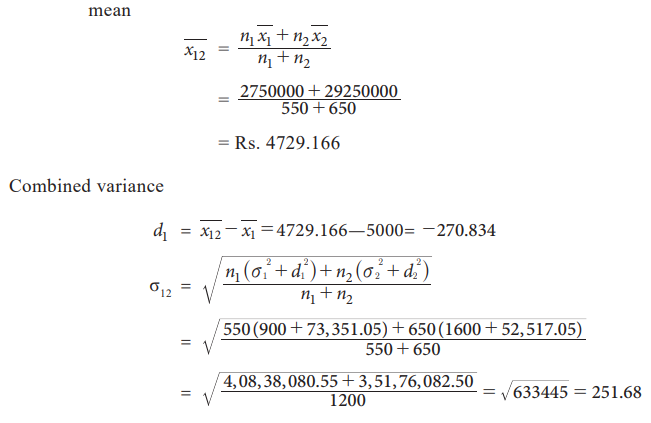Home | | Statistics 11th std | Combined Mean and Combined Standard Deviation

# Combined Mean and Combined Standard Deviation

Combined arithmetic mean can be computed if we know the mean and number of items in each group of the data.

Combined Mean and Combined Standard Deviation

Combined arithmetic mean can be computed if we know the mean and number of items in each group of the data.1,2, , σ1, σ2 are mean and standard deviation of two data sets having n1 and n2 as number of elements respectively.### Example 6.11

From the analysis of monthly wages paid to employees in two service organizations X and Y, the following results were obtainedi. Which organization pays a larger amount as monthly wages?

ii. In which organization is there greater variability in individual wages of all the wage earners taken together?

### Solution:

i. For finding out which organization X or Y pays larger amount of monthly wages, we have to compare the total wages:

Total wage bill paid monthly by X and Y isOrganization Y pays a larger amount as monthly wages as compared to organization X.

ii. For calculating the combined variance, we will first calculate the combined meanStudy Material, Lecturing Notes, Assignment, Reference, Wiki description explanation, brief detail
11th Statistics : Chapter 6 : Measures of Dispersion : Combined Mean and Combined Standard Deviation |